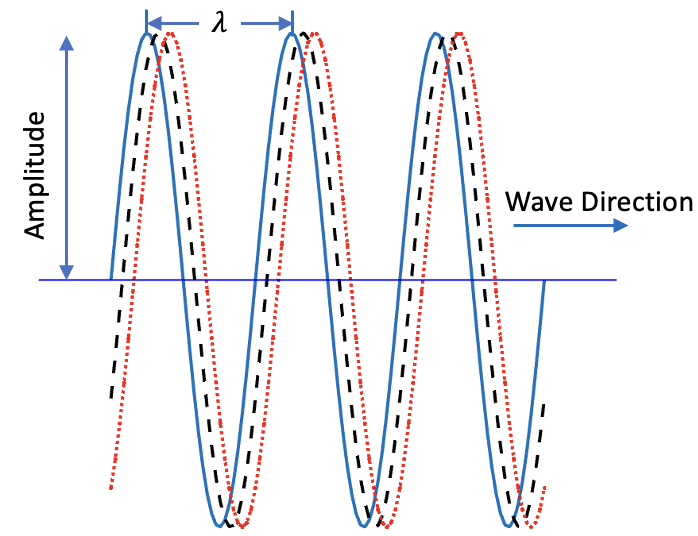## Section14.1Sinusoidal Waves

When a string is shaken sinusoidally, i.e., it is vibrated such that the oscillations are sine or cosine function of time, the wave propagated in the string also has sinusoidal shape as illustrated in Figure 14.1.1. The period of the wave in space is called its wavelength, and it is usually denoted by the Greek letter $\lambda$ (lambda). The absolute value of the displacement of the disturbance on either side of the equilibrium is called amplitude, usually denoted by letter $A\text{.}$ Note that amplitude is not the entire range of displacement but half of that.Figure 14.1.1. Traveling wave on a string. Figure shows wave at three instants of time. The repeating distance, denoted by $\lambda$ is the wavelength, the absolute value of the displacement of the disturbance on either side of the equilibrium is called amplitude, and the time it takes the wave to cover a wavelength is its period, which we denote by $T\text{.}$ The ratio $\lambda/T$ is the wave speed, which is also called phase velocity in the case of sinusoidal waves.

As Figure 14.1.1 shows, the moving wave will move with some speed $v\text{,}$ which we call wave speed. In the case of sinusoidal waves, wave speed is also called phase velocity. We will encounter another velocity associated with pulses or nonsinusoidal waves called group velocity. The wave in Figure 14.1.1 will travel a distance of the wavelength in some time $T\text{,}$ which is called period of the wave. That is,

\begin{equation} vT = \lambda.\label{eq-wave-fundametal-equation-1}\tag{14.1.1} \end{equation}

The inverse of the period is called frequency or cylce frequency or ordinary frequency of the wave. We will denote frequency by letter $f$ although sometimes it is also denoted by the Greek letter $\nu$ (nu).

\begin{equation} f = \frac{1}{T}.\tag{14.1.2} \end{equation}

In terms of frequency, Eq. (14.1.1)> takes the following form, called the fundamental equation of waves.

\begin{equation} v = \lambda\, f.\label{eq-wave-fundametal-equation-2}\tag{14.1.3} \end{equation}

### Subsection14.1.1Mathematical Description of Wave

Let string in Figure 14.1.1 be along $x$ axis and oscillate along the $y$ axis with frequency $f\text{.}$ Let $y(x,t)$ denote the displacement of string particle at coordinate $x$ at time $t\text{,}$ i.e., let the particle be at $(x,y)$ at instant t. Then, we can write the sinusoidal wave moving towards the positive $x$axis by

\begin{equation} y(x,t) = A\, \cos\left(\dfrac{2\pi}{\lambda} x - 2\pi f t + \phi\right),\tag{14.1.4} \end{equation}

where $\phi$ is a constant, called the phase constant. If diaplacement at origin at time $t=0$ is equal to the ampitude $A\text{,}$ then phase constant will be $\phi=0\text{.}$ We will usually take this to be the case.

\begin{equation} y(x,t) = A\, \cos\left(\dfrac{2\pi}{\lambda} x - 2\pi f t\right).\tag{14.1.5} \end{equation}

Rather than write $2\pi$ all over the place, we often write this using angular quantities by replacing frequency by angular frequency, $\omega\text{,}$

\begin{equation} \omega = 2\pi f,\tag{14.1.6} \end{equation}

and inverse wavelength by wave number, $k\text{,}$ defined by

\begin{equation} k = \frac{2\pi}{\lambda}.\tag{14.1.7} \end{equation}

the speed of the wave in terms of angular quantities will be

\begin{equation} v = f\lambda = \frac{\omega}{2\pi}\times \frac{2\pi}{k} = \frac{\omega}{k}.\tag{14.1.8} \end{equation}

Using angular variables, the sinusoidal wave moving to the right is given by

\begin{equation} y(x,t) = A\, \cos\left(k x - \omega t\right).\tag{14.1.9} \end{equation}

A wave moving towards left will have both $x$ and $t$ same sign.

\begin{equation} y(x,t) = A\, \cos\left(k x + \omega t\right).\tag{14.1.10} \end{equation}

The sound produced by a speaker is given by the following pressure difference, $\Delta p\text{,}$ in air.

\begin{equation*} \Delta p = (10 \text{ Pa})\, \cos(k x + 6000\, t + \pi), \end{equation*}

where $x$ is in meters and $t$ in seconds. Suppose the speed of this wave is $343\text{ m/s}\text{.}$ find the wavelength of the sound wave.

(a) What is the amplitude of the wave?

(b) What is the frequency of the wave?

(c) What is the wavelength of the wave?

Hint

The $\Delta p$ is just a sinusoidal wave.

(a) $10\text{ Pa}\text{,}$ (b) $954.9\text{ Hz}\text{,}$ (c) $35.9\text{ cm}\text{.}$

Solution

(a) Here $\Delta p$ is actually a sinusoidal wave. In the text, we used the symbol $y$ for the wave, but here, it is $\Delta p\text{.}$ The amplitude is just the number multiplying the oscillating part.

\begin{equation*} \text{Amplitude} = 10\text{ Pa}. \end{equation*}

(b) We can read of angular frequency $\omega$ from the number that multiplies $t$ in the argument of coine, and then obtained frequency $f$ from it.

\begin{equation*} f = \frac{\omega}{2\pi} = \frac{6000}{2\pi} = 954.9\text{ Hz}. \end{equation*}

I have used $\text{Hz}$ for the unit since it is regular cycle per second, and not the angular cycle.

(c) If we knew the wavenumber $k\text{,}$ we will get $\lambda$ from it. But, since we don't know $k$ yet, but we do know $v$ and $f\text{,}$ therefore, we will use the fundamental equation.

\begin{equation*} \lambda = \frac{v}{f} = \frac{343}{954.9} = 0.359\text{ m} = 35.9\text{ cm}. \end{equation*}